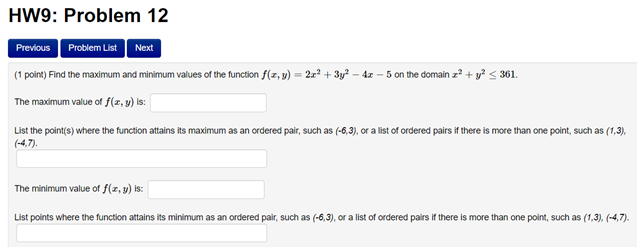# Question HW9: Problem 12 Previous Problem List Next (1 point) Find the maximum and minimum values of the function f(x,y) = 2x + 3y? - 43 - 5 on the domain 2? + y2 < 361. The maximum value of f(x,y) is: List the point(s) where the function attains its maximum as an ordered pair, such as (-6,3), or a list of ordered pairs if there is more than one point, such as (1,3). (-4,7). The minimum value of f(x,y) is: List points where the function attains its minimum as an ordered pair, such as (-6,3), or a list of ordered pairs if there is more than one point, such as (1,3), (-4,7).